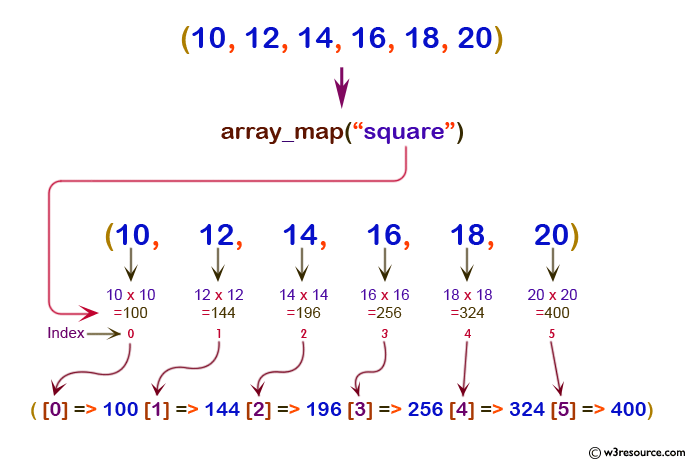# PHP: array_map() function

## PHP: Applies the callback to the elements of the given arrays

The array_map() function sends each value of an array to a user defined function and gets an array with new values applied by the user defined function.

Version:

(PHP 4 and above)

Syntax:

`array_map(user-supplied-function, array1, array2, ...)`

Parameters:

Name Description Required /
Optional
Type
user_function The user defined function to run for each element in each array. Required Array
array1 Specifies an array to run through the user defined function Required Array
array2.... Specifies an array to run through the user defined function Optional Array

Return value:

An array containing all the elements of array1 after applying the user_function() to each one.

Value Type: Array

Example:

``````<?php
function square(\$x)
{
return(\$x * \$x);
}
\$a = array(10,12,14,16,18,20);
\$b = array_map("square",\$a);
print_r(\$b);
?>
``````

Output:

`Array(  => 100  => 144  => 196  => 256  => 324  => 400)`

Pictorial Presentation:View the example in the browser

Practice here online :

PHP Function Reference

Previous: array_keys
Next: array_merge_ recursive

﻿

## PHP: Tips of the Day

PHP: Extract numbers from a string

```\$str = 'In My Cart : 11 12 items';
preg_match_all('!\d+!', \$str, \$matches);
print_r(\$matches);
```